# 第十三节、SURF特征提取算法

SURF(Speeded Up Robust Features)算法改进了特征了提取和描述方式，用一种更为高效的方式完成特征点的提取和描述。

### 一 使用快速Hessian算法和SURF来提取和检测特征

SURF特征检测算法由Herbert Lowe于2006年发表，该算法比SIFT算法快好几倍，它吸收了SIFT算法的思想。

SURF算法采用快速Hessian算法检测关键点，而SURF算子会通过一个特征向量来描述关键点周围区域的情况。这和SIFT算法很像，SIFT算法分别采用DoG和SIFT算子来检测关键点和提取关键点的描述符。

#### 1.1 示例

# -*- coding: utf-8 -*-
"""
Created on Fri Aug 24 20:09:32 2018

@author: lenovo
"""

# -*- coding: utf-8 -*-
"""
Created on Wed Aug 22 16:53:16 2018

@author: lenovo
"""

'''
SURF算法
'''
import cv2
import numpy as np

img = cv2.resize(img,dsize=(600,400))
#转换为灰度图像
gray = cv2.cvtColor(img,cv2.COLOR_BGR2GRAY)
#创建一个SURF对象
surf = cv2.xfeatures2d.SURF_create(20000)
#SIFT对象会使用Hessian算法检测关键点，并且对每个关键点周围的区域计算特征向量。该函数返回关键点的信息和描述符
keypoints,descriptor = surf.detectAndCompute(gray,None)
print(type(keypoints),len(keypoints),keypoints)
print(descriptor.shape)
#在图像上绘制关键点
img = cv2.drawKeypoints(image=img,keypoints = keypoints,outImage=img,color=(255,0,255),flags=cv2.DRAW_MATCHES_FLAGS_DRAW_RICH_KEYPOINTS)
#显示图像
cv2.imshow('surf_keypoints',img)
cv2.waitKey(0)
cv2.destroyAllWindows()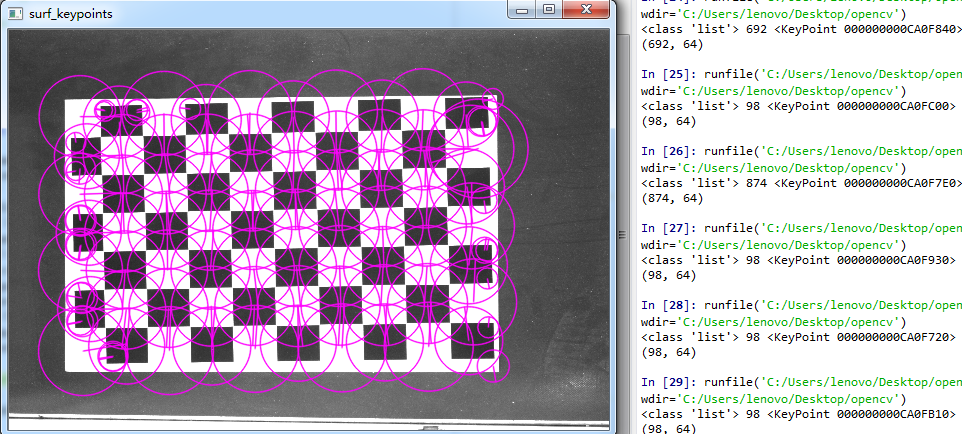### 二 SURF算法原理

#### 2.1 SURF特征检测的步骤

1. 尺度空间的极值检测：搜索所有尺度空间上的图像，通过Hessian来识别潜在的对尺度和选择不变的兴趣点。
2. 特征点过滤并进行精确定位。
3. 特征方向赋值：统计特征点圆形邻域内的Harr小波特征。即在60度扇形内，每次将60度扇形区域旋转0.2弧度进行统计，将值最大的那个扇形的方向作为该特征点的主方向。
4. 特征点描述：沿着特征点主方向周围的邻域内，取$4×4$个矩形小区域，统计每个小区域的Haar特征，然后每个区域得到一个4维的特征向量。一个特征点共有64维的特征向量作为SURF特征的描述子。

#### 2.2 构建Hessian(黑塞矩阵)

$$H(I(x,y))=\begin{bmatrix} \frac{\partial^2I}{\partial{x^2}} & \frac{\partial^2I}{\partial{x}\partial{y}} \\ \frac{\partial^2I}{\partial{x}\partial{y}} & \frac{\partial^2I}{\partial{y^2}} \end{bmatrix}$$

H矩阵的判别式是：

$$Det(H)=\frac{\partial^2I}{\partial{x^2}}*\frac{\partial^2I}{\partial{y^2}}-\frac{\partial^2I}{\partial{x}\partial{y}} * \frac{\partial^2I}{\partial{x}\partial{y}}$$

$$H(x,y,\sigma)=\begin{bmatrix} L_{xx}(x,y,\sigma) & L_{xy}(x,y,\sigma) \\ L_{xy}(x,y,\sigma) & L_{yy}(x,y,\sigma) \end{bmatrix}$$

$$L_x=L(x+1,y)-L(x,y)$$

$$L_{xx}=[L(x+1,y)-L(x,y)]-[L(x,y)-L(x-1,y)]$$

$$=L(x+1,y)+L(x-1,y)-2L(x,y)$$

$$Det(H)=L_{xx}*L_{yy}-L_{xy}*L_{xy}$$

$$Det(H)=L_{xx}*L_{yy}-(0.9*L_{xy})^2$$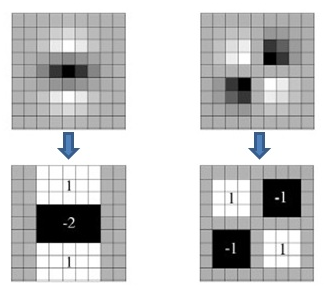#### 2.3 构造尺度空间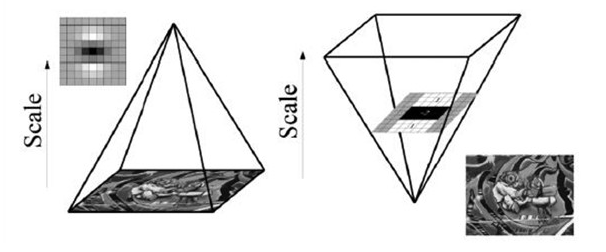#### 2.4 特征点过滤并进行精确定位

SURF特征点的定位过程和SIFT算法一致，将经过Hessian矩阵处理的每个像素点(即获得每个像素点Hessian矩阵的判别式值)与其图像域（相同大小的图像）和尺度域（相邻的尺度空间）的所有相邻点进行比较，当其大于（或者小于）所有相邻点时，该点就是极值点。如图所示，中间的检测点要和其所在图像的$3×3$邻域8个像素点，以及其相邻的上下两层$3×3$邻域18个像素点，共26个像素点进行比较。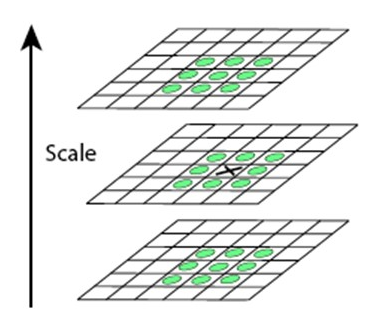#### 2.5 计算特征点主方向

SIFT算法特征点的主方向是采用在特征点邻域内统计其梯度直方图，横轴是梯度方向的角度纵轴是梯度方向对应梯度幅值的累加，取直方图bin最大的以及超过最大80%的那些方向作为特征点的主方向。Harr特征的具体内容可以参考第九节、人脸检测之Haar分类器

#### 2.6 生成特征描述

SURF算法中，也是提取特征点周围$4×4$个矩形区域块，但是所取得矩形区域方向是沿着特征点的主方向，而不是像SIFT算法一样，经过旋转$\theta$角度。每个子区域统计25个像素点水平方向和垂直方向的Haar小波特征，这里的水平和垂直方向都是相对主方向而言的。该Harr小波特征为水平方向值之和、垂直方向值之和、水平方向值绝对值之和以及垂直方向绝对之和4个方向。该过程示意图如下：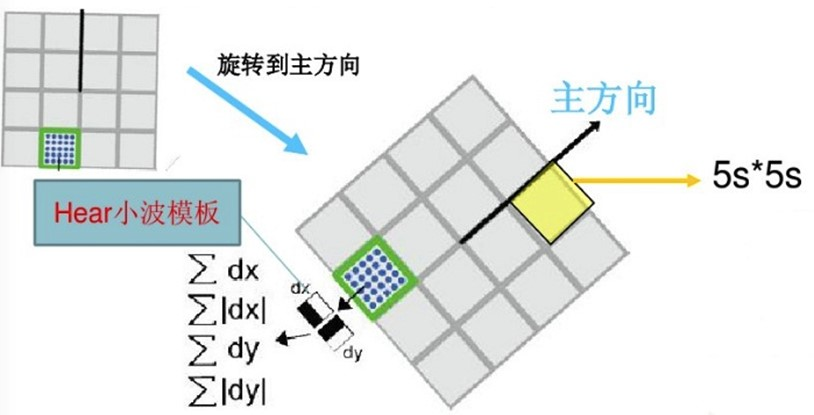### 三 特征点匹配

# -*- coding: utf-8 -*-
"""
Created on Fri Aug 24 20:09:32 2018

@author: lenovo
"""

# -*- coding: utf-8 -*-
"""
Created on Wed Aug 22 16:53:16 2018

@author: lenovo
"""

'''
SURF算法
'''
import cv2
import numpy as np

'''1、加载图片'''
img1 = cv2.resize(img1,dsize=(600,400))
img2 = cv2.resize(img2,dsize=(600,400))
image1 = img1.copy()
image2 = img2.copy()

'''2、提取特征点'''
#创建一个SURF对象
surf = cv2.xfeatures2d.SURF_create(25000)
#SIFT对象会使用Hessian算法检测关键点，并且对每个关键点周围的区域计算特征向量。该函数返回关键点的信息和描述符
keypoints1,descriptor1 = surf.detectAndCompute(image1,None)
keypoints2,descriptor2 = surf.detectAndCompute(image2,None)
print('descriptor1:',descriptor1.shape,'descriptor2',descriptor2.shape)
#在图像上绘制关键点
image1 = cv2.drawKeypoints(image=image1,keypoints = keypoints1,outImage=image1,color=(255,0,255),flags=cv2.DRAW_MATCHES_FLAGS_DRAW_RICH_KEYPOINTS)
image2 = cv2.drawKeypoints(image=image2,keypoints = keypoints2,outImage=image2,color=(255,0,255),flags=cv2.DRAW_MATCHES_FLAGS_DRAW_RICH_KEYPOINTS)
#显示图像
cv2.imshow('surf_keypoints1',image1)
cv2.imshow('surf_keypoints2',image2)
cv2.waitKey(20)

'''3、特征点匹配'''
matcher = cv2.FlannBasedMatcher()
matchePoints = matcher.match(descriptor1,descriptor2)
print(type(matchePoints),len(matchePoints),matchePoints)

#提取强匹配特征点
minMatch = 1
maxMatch = 0
for i in range(len(matchePoints)):
if  minMatch > matchePoints[i].distance:
minMatch = matchePoints[i].distance
if  maxMatch < matchePoints[i].distance:
maxMatch = matchePoints[i].distance
print('最佳匹配值是:',minMatch)
print('最差匹配值是:',maxMatch)

#获取排雷在前边的几个最优匹配结果
goodMatchePoints = []
for i in range(len(matchePoints)):
if matchePoints[i].distance < minMatch + (maxMatch-minMatch)/16:
goodMatchePoints.append(matchePoints[i])

#绘制最优匹配点
outImg = None
outImg = cv2.drawMatches(img1,keypoints1,img2,keypoints2,goodMatchePoints,outImg,matchColor=(0,255,0),flags=cv2.DRAW_MATCHES_FLAGS_DEFAULT)
cv2.imshow('matche',outImg)
cv2.waitKey(0)
cv2.destroyAllWindows()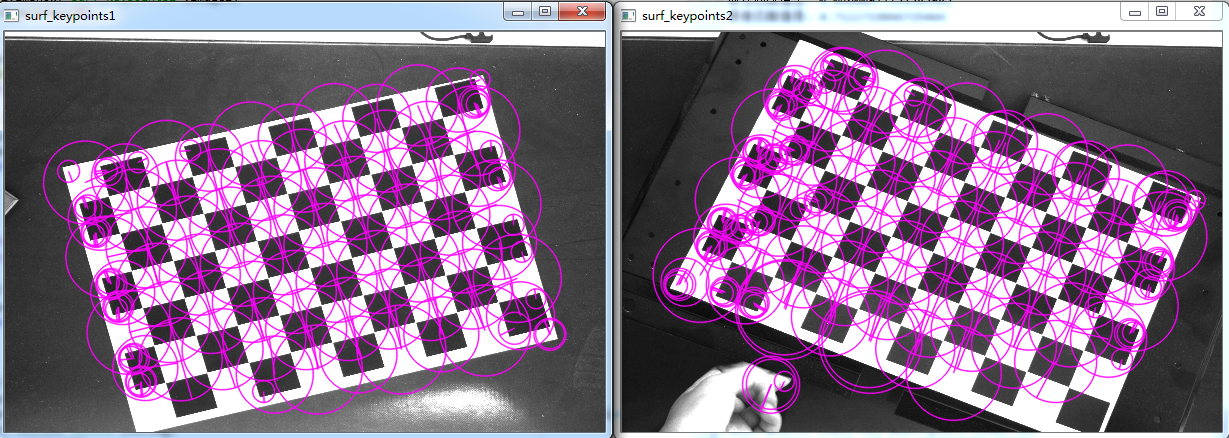# -*- coding: utf-8 -*-
"""
Created on Fri Aug 24 20:09:32 2018

@author: lenovo
"""

# -*- coding: utf-8 -*-
"""
Created on Wed Aug 22 16:53:16 2018

@author: lenovo
"""

'''
SURF算法
'''
import cv2

'''1、加载图片'''
img1 = cv2.resize(img1,dsize=(600,400))
gray1 = cv2.cvtColor(img1,cv2.COLOR_BGR2GRAY)
img2 = cv2.resize(img2,dsize=(600,400))
gray2 = cv2.cvtColor(img2,cv2.COLOR_BGR2GRAY)
image1 = gray1.copy()
image2 = gray2.copy()

'''2、提取特征点'''
#创建一个SURF对象
surf = cv2.xfeatures2d.SURF_create(10000)
#SIFT对象会使用Hessian算法检测关键点，并且对每个关键点周围的区域计算特征向量。该函数返回关键点的信息和描述符
keypoints1,descriptor1 = surf.detectAndCompute(image1,None)
keypoints2,descriptor2 = surf.detectAndCompute(image2,None)
print('descriptor1:',descriptor1.shape,'descriptor2',descriptor2.shape)
#在图像上绘制关键点
image1 = cv2.drawKeypoints(image=image1,keypoints = keypoints1,outImage=image1,color=(255,0,255),flags=cv2.DRAW_MATCHES_FLAGS_DRAW_RICH_KEYPOINTS)
image2 = cv2.drawKeypoints(image=image2,keypoints = keypoints2,outImage=image2,color=(255,0,255),flags=cv2.DRAW_MATCHES_FLAGS_DRAW_RICH_KEYPOINTS)
#显示图像
cv2.imshow('surf_keypoints1',image1)
cv2.imshow('surf_keypoints2',image2)
cv2.waitKey(20)

'''3、特征点匹配'''
matcher = cv2.FlannBasedMatcher()
matchePoints = matcher.match(descriptor1,descriptor2)
print(type(matchePoints),len(matchePoints),matchePoints)

#提取强匹配特征点
minMatch = 1
maxMatch = 0
for i in range(len(matchePoints)):
if  minMatch > matchePoints[i].distance:
minMatch = matchePoints[i].distance
if  maxMatch < matchePoints[i].distance:
maxMatch = matchePoints[i].distance
print('最佳匹配值是:',minMatch)
print('最差匹配值是:',maxMatch)

#获取排雷在前边的几个最优匹配结果
goodMatchePoints = []
for i in range(len(matchePoints)):
if matchePoints[i].distance < minMatch + (maxMatch-minMatch)/4:
goodMatchePoints.append(matchePoints[i])

#绘制最优匹配点
outImg = None
outImg = cv2.drawMatches(img1,keypoints1,img2,keypoints2,goodMatchePoints,outImg,matchColor=(0,255,0),flags=cv2.DRAW_MATCHES_FLAGS_DEFAULT)
cv2.imshow('matche',outImg)
cv2.waitKey(0)
cv2.destroyAllWindows()

### 四 代码下载

图像识别基本算法之SURF

SURF算法

SURF算法与源码分析、上

SURF算法与源码分析、下

SURF原理及源码解析(C++)

posted @ 2018-08-24 22:08  大奥特曼打小怪兽  阅读(18306)  评论(0编辑  收藏  举报